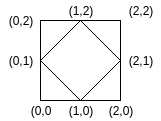# Count the number of rhombi possible inside a rectangle of given size

Given a rectangle of height H and width W which has the bottom left corner at (0, 0). The task is to count the number of distinct Rhombi that have all points inside or on the border of the rectangle satisfying the following conditions exists:

• Have non-zero area.
• Have diagonals parallel to the x and y axes.
• Have integer coordinates.

Examples:

Input: H = 2, W = 2
Output:
There is only one rhombus possible with coordinates (0, 1), (1, 0), (2, 1) and (1, 2).Input: H = 4, W = 4
Output: 16

Approach: Since the diagonals are parallel to the axis, let’s try fixing the diagonals and creating rhombi on them. For the rhombus to have integer coordinates, the length of the diagonals must be even. Let’s fix the length of the diagonals to i and j, the number of rhombi we can form with these diagonal lengths inside the rectangle would be (H – i + 1) * (W – j + 1). Thus, we iterate over all possible values of i and j and update the count.
Below is the implementation of the above approach:

## C++

 `// C++ implementation of the approach` `#include ` `using` `namespace` `std;`   `// Function to return the count of rhombi possible` `long` `long` `countRhombi(``int` `h, ``int` `w)` `{` `    ``long` `long` `ct = 0;`   `    ``// All possible diagonal lengths` `    ``for` `(``int` `i = 2; i <= h; i += 2)` `        ``for` `(``int` `j = 2; j <= w; j += 2)`   `            ``// Update rhombi possible with` `            ``// the current diagonal lengths` `            ``ct += (h - i + 1) * (w - j + 1);`   `    ``// Return the total count` `    ``// of rhombi possible` `    ``return` `ct;` `}`   `// Driver code` `int` `main()` `{` `    ``int` `h = 2, w = 2;`   `    ``cout << countRhombi(h, w);`   `    ``return` `0;` `}`

## Java

 `// Java implementation of the approach` `import` `java.io.*;`   `class` `GFG ` `{` `    `  `// Function to return the count of rhombi possible` `static` `int` `countRhombi(``int` `h, ``int` `w)` `{` `    ``int` `ct = ``0``;`   `    ``// All possible diagonal lengths` `    ``for` `(``int` `i = ``2``; i <= h; i += ``2``)` `        ``for` `(``int` `j = ``2``; j <= w; j += ``2``)`   `            ``// Update rhombi possible with` `            ``// the current diagonal lengths` `            ``ct += (h - i + ``1``) * (w - j + ``1``);`   `    ``// Return the total count` `    ``// of rhombi possible` `    ``return` `ct;` `}`   `    ``// Driver code` `    ``public` `static` `void` `main (String[] args) ` `    ``{` `    ``int` `h = ``2``, w = ``2``;` `    ``System.out.println (countRhombi(h, w));` `    ``}` `}`   `// This code is contributed by jit_t`

## Python 3

 `# Python 3 implementation of the approach`   `# Function to return the count of ` `# rhombi possible` `def` `countRhombi(h, w):`   `    ``ct ``=` `0``;`   `    ``# All possible diagonal lengths` `    ``for` `i ``in` `range``(``2``, h ``+` `1``, ``2``):` `        ``for` `j ``in` `range``(``2``, w ``+` `1``, ``2``):`   `            ``# Update rhombi possible with` `            ``# the current diagonal lengths` `            ``ct ``+``=` `(h ``-` `i ``+` `1``) ``*` `(w ``-` `j ``+` `1``)`   `    ``# Return the total count` `    ``# of rhombi possible` `    ``return` `ct`   `# Driver code` `if` `__name__ ``=``=` `"__main__"``:`   `    ``h ``=` `2` `    ``w ``=` `2`   `    ``print``(countRhombi(h, w))`   `# This code is contributed by ita_c`

## C#

 `// C# program to find the frequency of ` `// minimum element in the array ` `using` `System;`   `class` `GFG ` `{ ` `        `  `    ``// Function to return the count` `    ``// of rhombi possible ` `    ``static` `int` `countRhombi(``int` `h, ``int` `w) ` `    ``{ ` `        ``int` `ct = 0; ` `    `  `        ``// All possible diagonal lengths ` `        ``for` `(``int` `i = 2; i <= h; i += 2) ` `            ``for` `(``int` `j = 2; j <= w; j += 2) ` `    `  `                ``// Update rhombi possible with ` `                ``// the current diagonal lengths ` `                ``ct += (h - i + 1) * (w - j + 1); ` `    `  `        ``// Return the total count ` `        ``// of rhombi possible ` `        ``return` `ct; ` `    ``} ` `    `  `    ``// Driver code ` `    ``public` `static` `void` `Main() ` `    ``{ ` `        ``int` `h = 2, w = 2; ` `        `  `        ``Console.WriteLine(countRhombi(h, w)); ` `    ``} ` `} `   `// This code is contributed by Ryuga `

## PHP

 ``

## Javascript

 ``

Output:

`1`

Time Complexity: O(H * W)

Auxiliary Space: O(1)

Feeling lost in the world of random DSA topics, wasting time without progress? It's time for a change! Join our DSA course, where we'll guide you on an exciting journey to master DSA efficiently and on schedule.
Ready to dive in? Explore our Free Demo Content and join our DSA course, trusted by over 100,000 geeks!

Previous
Next